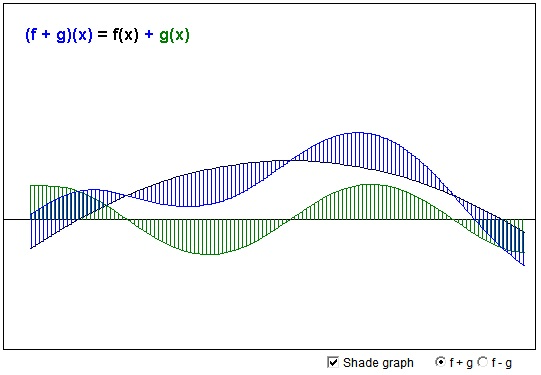# Addition and Subtraction of Functions

Addition of functions is a pointwise operation:

$(f + g)(x) = f(x) + g(x).$

Subtraction is defined similarly as

$(f - g)(x) = f(x) - g(x).$

In the applet below, the graphs of both $f\,$ and $g\,$ could be dragged up and down at any point. This will automatically change the result of the operation. The graph of $f\,$ is depicted in black and that of $g\,$ in green. For the sum $(f + g),\,$ I use blue, and for the difference $(f - g)\,$ red color. Thus of the three graphs only two -- the black and the green -- are draggable.

### If you are reading this, your browser is not set to run Java applets. Try IE11 or Safari and declare the site https://www.cut-the-knot.org as trusted in the Java setup.What if applet does not run?• What Is Line?
• Functions, what are they?
• Cartesian Coordinate System
• Function, Derivative and Integral
• Graph of a Polynomial of arbitrary degree
• Graph of a Polynomial Defined by Its Roots
• Inflection Points of Fourth Degree Polynomials
• Lagrange Interpolation (an Interactive Gizmo)
• Equations of a Straight Line
• Taylor Series Approximation to Cosine
• Taylor Series Approximation to Cosine
• Linear Function with Coefficients in Arithmetic Progression
• Sine And Cosine Are Continuous Functions
•